# ICSE Class 8 Maths Selina Solutions Chapter 7 Percent and Percentage

Per cent is derived from the Latin word ‘per centum’ meaning ‘per hundred’. It is represented by the symbol % and means 100. If we say 1% that means 1 out of 100 or one part of a complete quantity. In chapter 7 of ICSE Class 8 Maths, you will learn all the concepts related to Percent and Percentage. Also, you will solve the slightly advanced problems related to the application of percentages in the exercise.

We have provided the ICSE Class 8 Maths Selina Solutions Chapter 7 Percent and Percentage in PDF format to help you in your studies. These questions are solved by the experts at BYJU’S with detailed explanation in simple language. In case you have missed the class then refer to this pdf to understand the problems. Click on the link below to download the PDF.

## Download ICSE Class 8 Maths Selina Solutions PDF for Chapter 7:-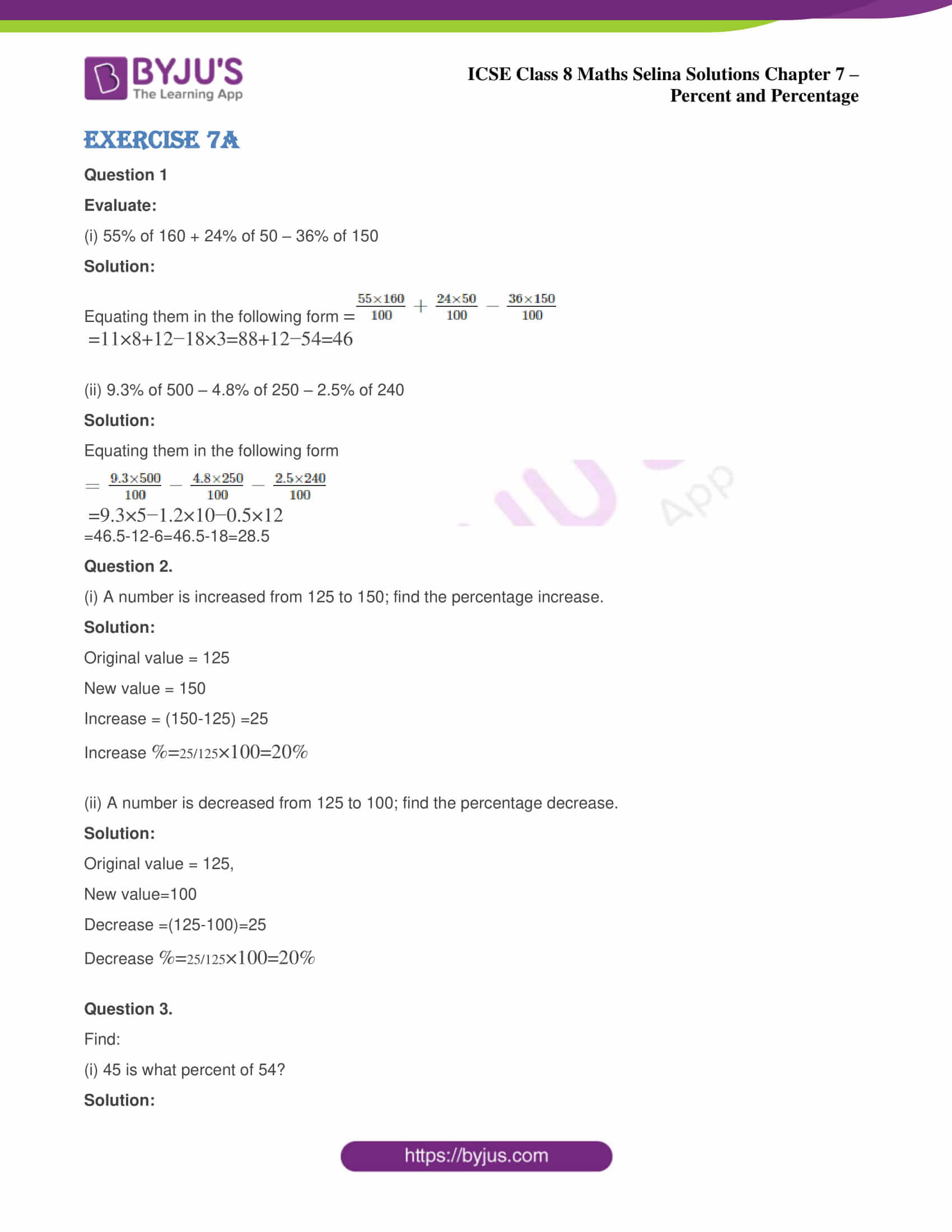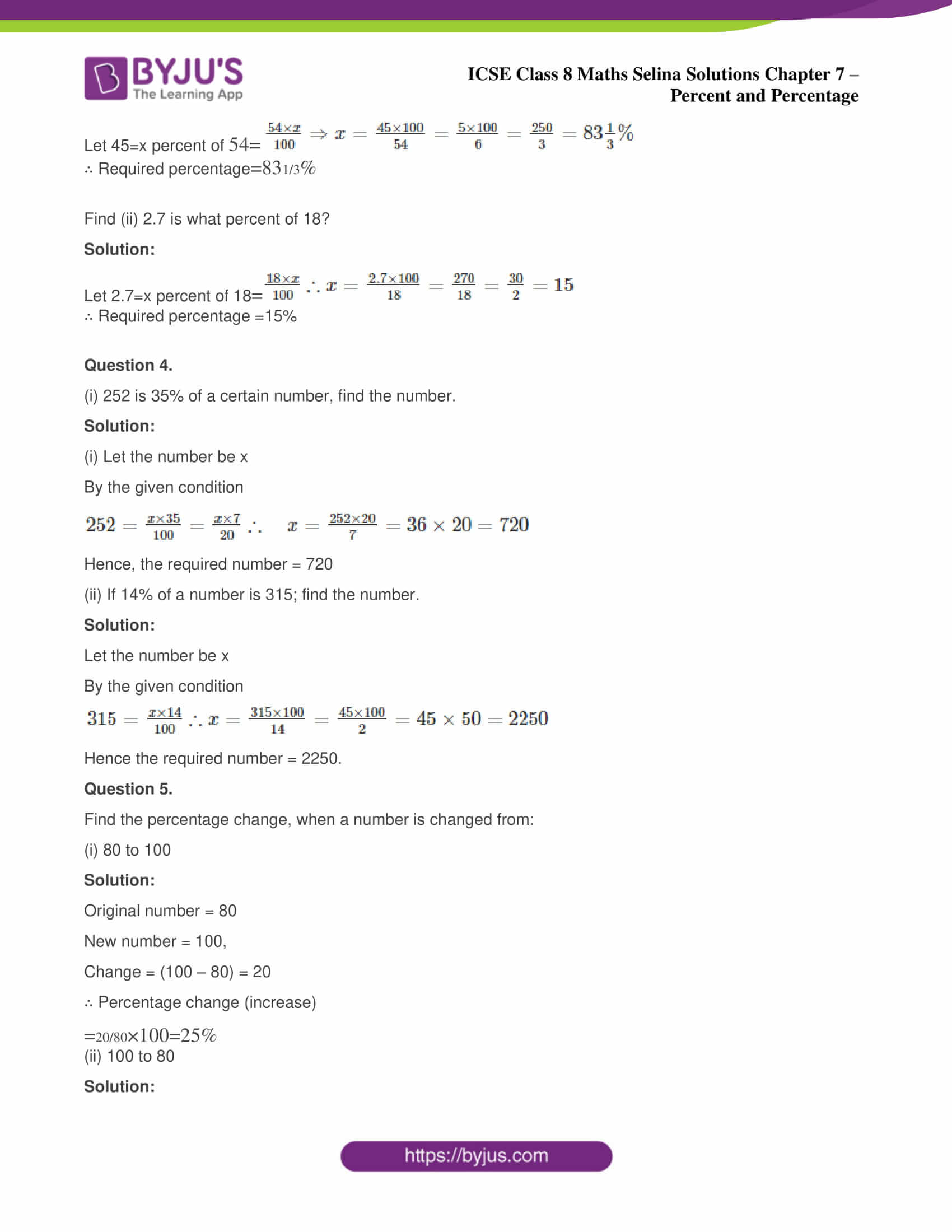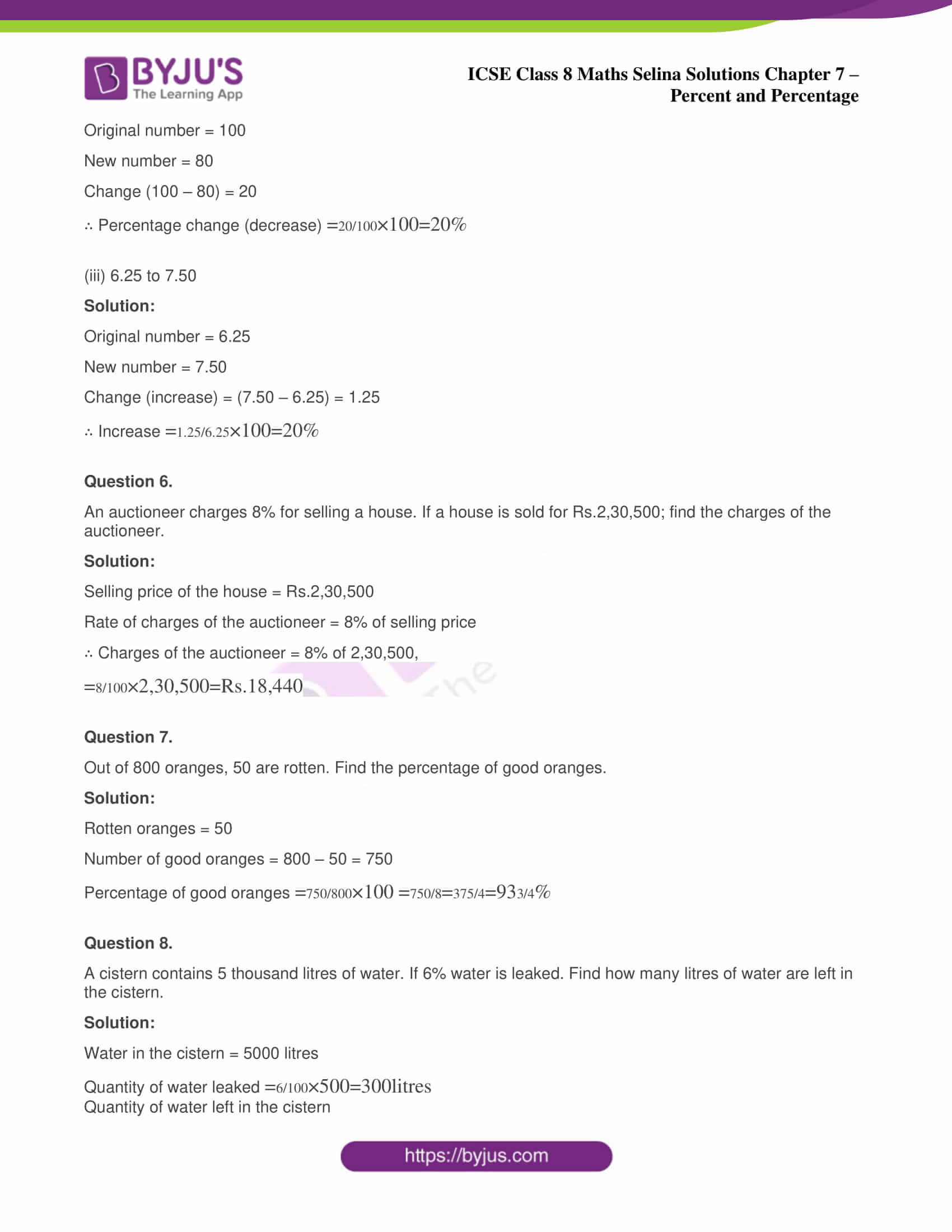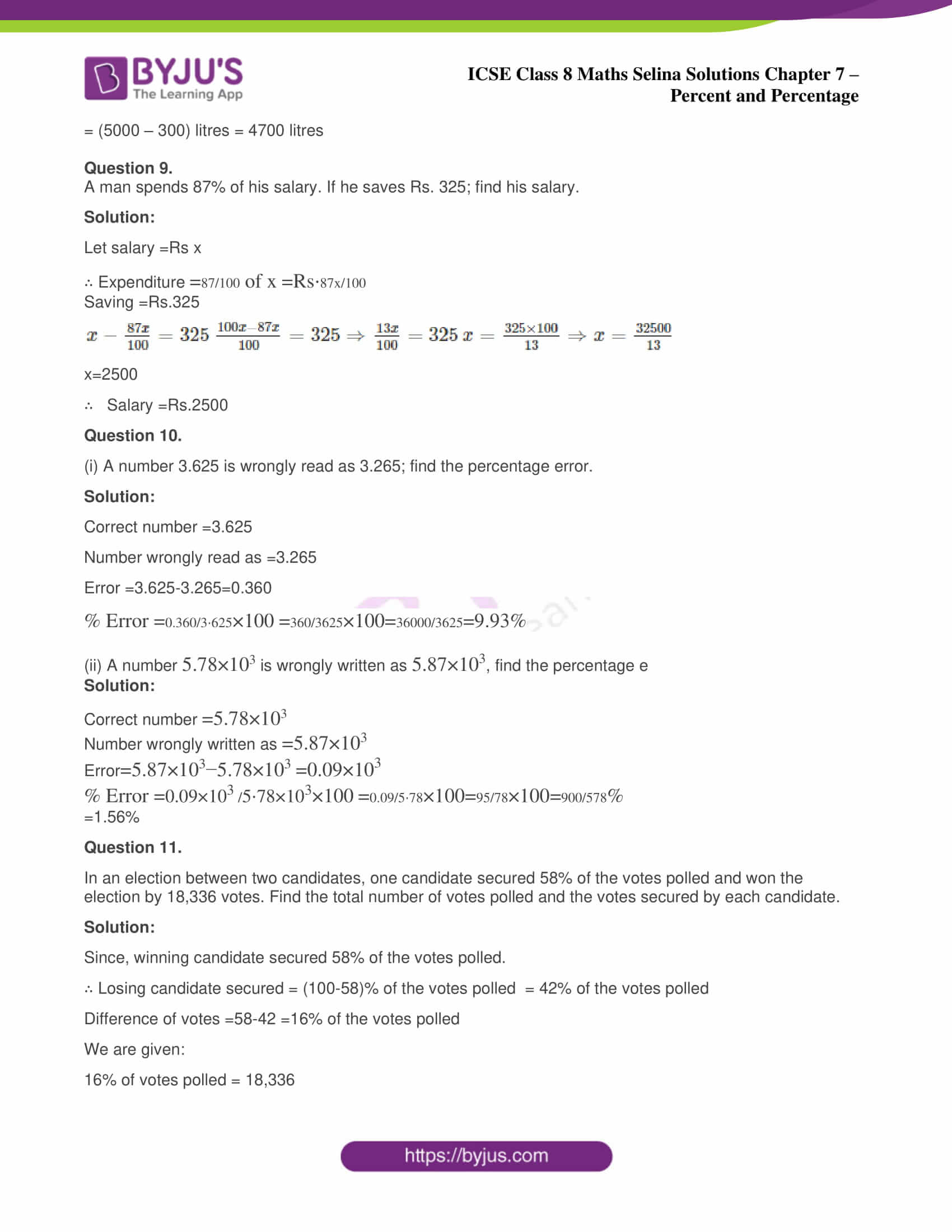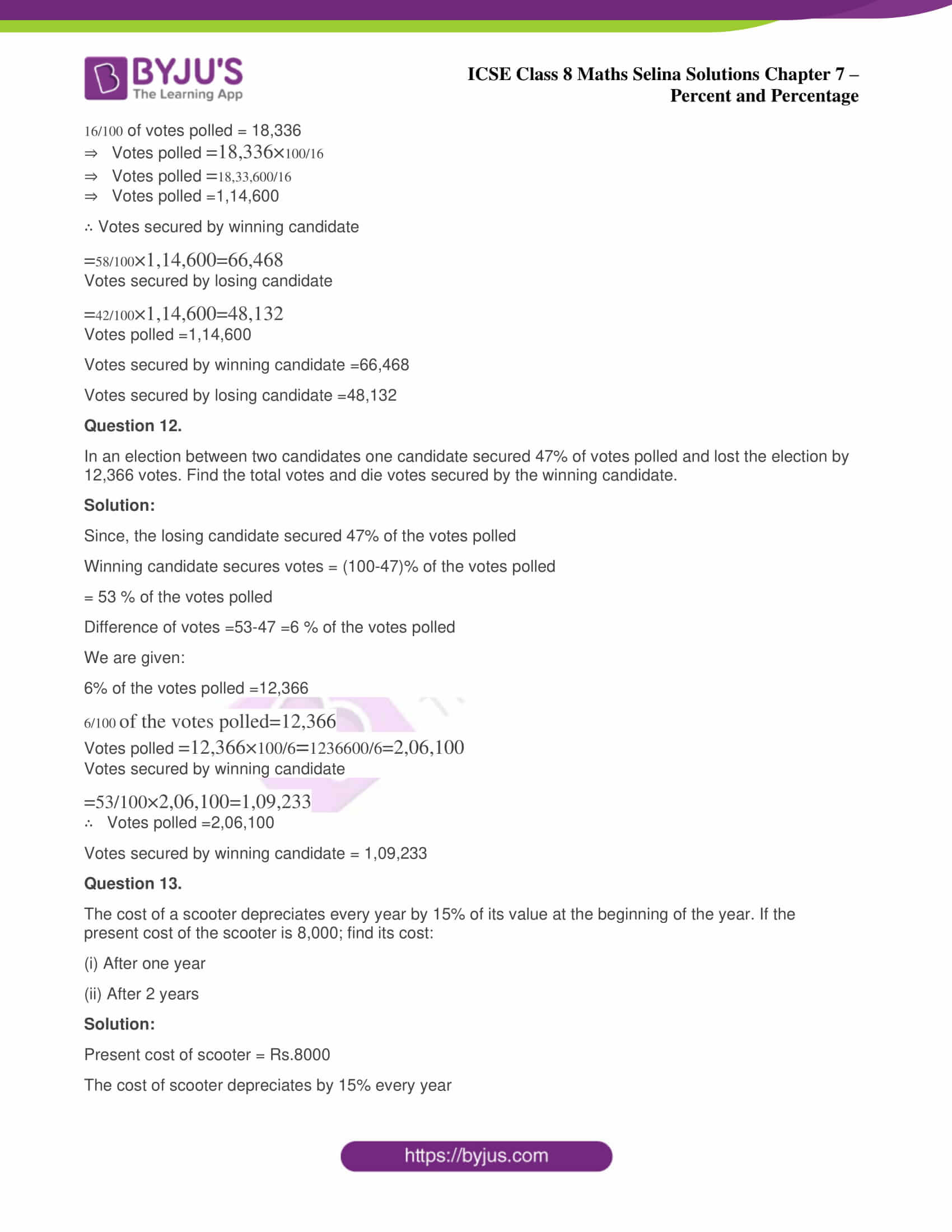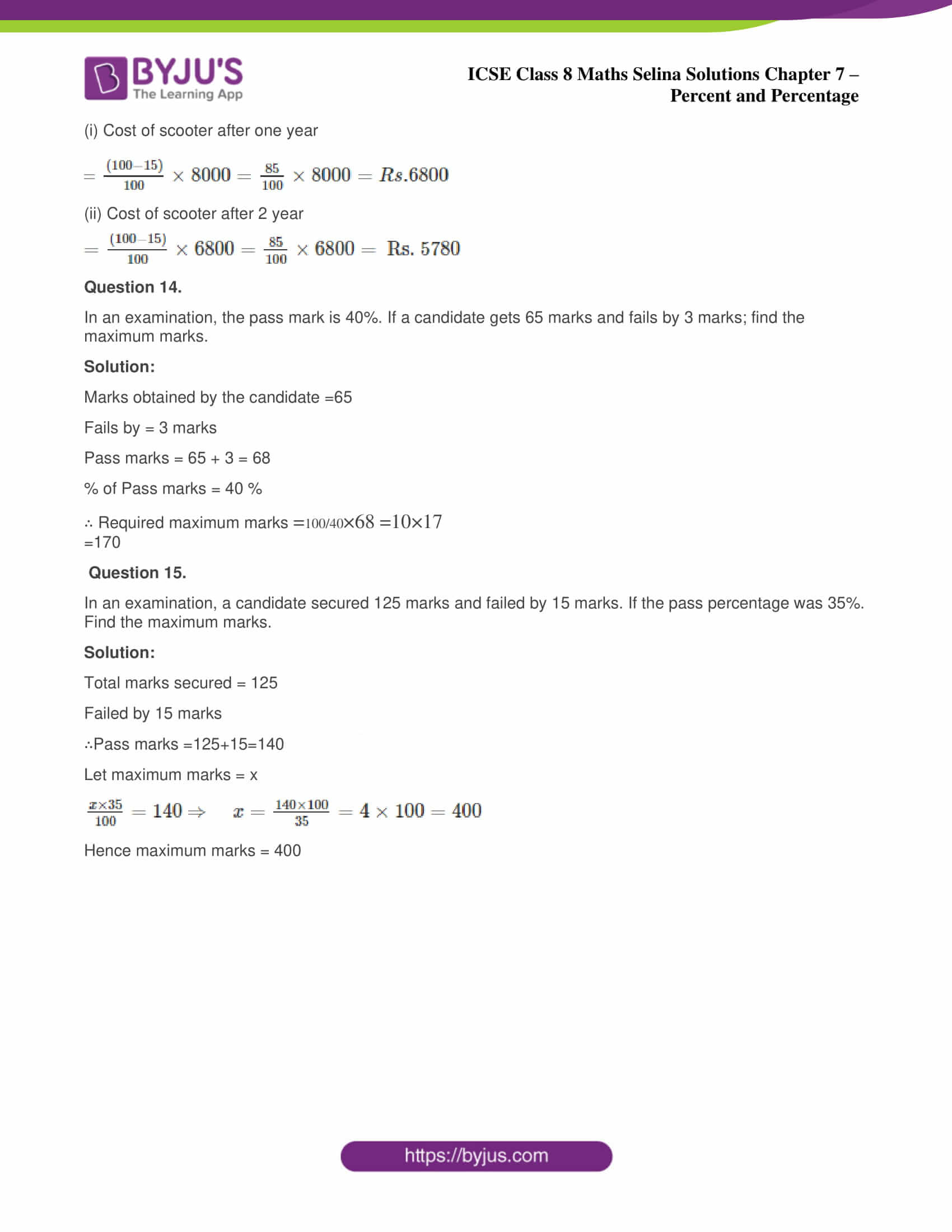ICSE Class 8 Maths Chapter 7 Percent and Percentage has only one exercise i.e 7 (A) consisting a total of 15 questions. The answers to all these questions have also been shown below;

### ICSE Class 8 Maths Selina Solutions Chapter 7 Percent and Percentage – Exercise 7 (A)

Question 1

Evaluate:

(i) 55% of 160 + 24% of 50 – 36% of 150

Solution:

Equating them in the following form

$$\begin{array}{l} =\frac{55 \times 160}{100}+\frac{24 \times 50}{100}-\frac{36 \times 150}{100} \end{array}$$
$$\begin{array}{l} =11 \times 8+12-18 \times 3=88+12-54=46\end{array}$$

(ii) 9.3% of 500 – 4.8% of 250 – 2.5% of 240

Solution:

Equating them in the following form

$$\begin{array}{l} =\frac{9.3 \times 500}{100}-\frac{4.8 \times 250}{100}-\frac{2.5 \times 240}{100}\end{array}$$
$$\begin{array}{l} =9.3 \times 5-1.2 \times 10-0.5 \times 12\end{array}$$

=46.5-12-6=46.5-18=28.5

Question 2.

(i) A number is increased from 125 to 150; find the percentage increase.

Solution:

Original value = 125

New value = 150

Increase = (150-125) =25

Increase

$$\begin{array}{l} \%=\frac{25}{125} \times 100=20 \% \end{array}$$

(ii) A number is decreased from 125 to 100; find the percentage decrease.

Solution:

Original value = 125,

New value=100

Decrease =(125-100)=25

Decrease

$$\begin{array}{l} \%=\frac{25}{125} \times 100=20 \%\end{array}$$

Question 3.

Find:

(i) 45 is what percent of 54?

Solution:

Let 45=x percent of

$$\begin{array}{l} 54=\frac{54 \times x}{100} \end{array}$$
$$\begin{array}{l} \Rightarrow x=\frac{45 \times 100}{54}=\frac{5 \times 100}{6}\end{array}$$
$$\begin{array}{l} =\frac{250}{3}=83 \frac{1}{3} \%\end{array}$$

∴ Required percentage

$$\begin{array}{l} =83 \frac{1}{3} \%\end{array}$$

Find (ii) 2.7 is what percent of 18?

Solution:

Let 2.7=x percent of 18

$$\begin{array}{l} =\frac{18 \times x}{100}\end{array}$$
$$\begin{array}{l} ∴ x=\frac{2.7 \times 100}{18}=\frac{270}{18}=\frac{30}{2}=15\end{array}$$

∴ Required percentage =15%

Question 4.

(i) 252 is 35% of a certain number, find the number.

Solution:

(i) Let the number be x

By the given condition

$$\begin{array}{l} 252=\frac{x \times 35}{100}=\frac{x \times 7}{20}\end{array}$$
$$\begin{array}{l} ∴ \quad x=\frac{252 \times 20}{7}=36 \times 20=720\end{array}$$

Hence, the required number = 720

(ii) If 14% of a number is 315; find the number.

Solution:

Let the number be x

By the given condition

$$\begin{array}{l} 315=\frac{x \times 14}{100}\end{array}$$
$$\begin{array}{l} ∴ x=\frac{315 \times 100}{14}=\frac{45 \times 100}{2}=45 \times 50=2250\end{array}$$

Hence the required number = 2250.

Question 5.

Find the percentage change, when a number is changed from:

(i) 80 to 100

Solution:

Original number = 80

New number = 100,

Change = (100 – 80) = 20

∴ Percentage change (increase)

$$\begin{array}{l} =\frac{20}{80} \times 100=25 \%\end{array}$$

(ii) 100 to 80

Solution:

Original number = 100

New number = 80

Change (100 – 80) = 20

∴ Percentage change (decrease)

$$\begin{array}{l} =\frac{20}{100} \times 100=20 \%\end{array}$$

(iii) 6.25 to 7.50

Solution:

Original number = 6.25

New number = 7.50

Change (increase) = (7.50 – 6.25) = 1.25

∴ Increase

$$\begin{array}{l} =\frac{1.25}{6.25} \times 100=20 \% \end{array}$$

Question 6.

An auctioneer charges 8% for selling a house. If a house is sold for Rs.2,30,500; find the charges of the auctioneer.

Solution:

Selling price of the house = Rs.2,30,500

Rate of charges of the auctioneer = 8% of selling price

∴ Charges of the auctioneer = 8% of 2,30,500,

$$\begin{array}{l} =\frac{8}{100} \times 2,30,500=R s .18,440\end{array}$$

Question 7.

Out of 800 oranges, 50 are rotten. Find the percentage of good oranges.

Solution:

Rotten oranges = 50

Number of good oranges = 800 – 50 = 750

Percentage of good oranges

$$\begin{array}{l} =\frac{750}{800} \times 100\end{array}$$
$$\begin{array}{l} =\frac{750}{8}=\frac{375}{4}=93 \frac{3}{4} \%\end{array}$$

Question 8.

A cistern contains 5 thousand liters of water. If 6% water is leaked. Find how many liters of water are left in the cistern.

Solution:

Water in the cistern = 5000 liters

Quantity of water leaked

$$\begin{array}{l} =\frac{6}{100} \times 500=300 liters\end{array}$$

Quantity of water left in the cistern

= (5000 – 300) liters = 4700 liters

Question 9.

A man spends 87% of his salary. If he saves Rs. 325; find his salary.

Solution:

Let salary =Rs x

∴ Expenditure

$$\begin{array}{l} =\frac{87}{100} \text { of } x\end{array}$$
$$\begin{array}{l} =\mathrm{Rs} \cdot \frac{87 \mathrm{x}}{100}\end{array}$$

Saving =Rs.325

$$\begin{array}{l} x-\frac{87 x}{100}=325\end{array}$$

$$\begin{array}{l} \frac{100 x-87 x}{100}=325 \Rightarrow \frac{13 x}{100}=325\end{array}$$

$$\begin{array}{l} x=\frac{325 \times 100}{13} \Rightarrow x=\frac{32500}{13}\end{array}$$

x=2500

∴   Salary =Rs.2500

Question 10.

(i) A number 3.625 is wrongly read as 3.265; find the percentage error.

Solution:

Correct number =3.625

Error =3.625-3.265=0.360

$$\begin{array}{l} \% \text { Error }=\frac{0.360}{3 \cdot 625} \times 100\end{array}$$
$$\begin{array}{l} =\frac{360}{3625} \times 100=\frac{36000}{3625}=9.93 \%\end{array}$$

(ii) A number

$$\begin{array}{l} 5.78 \times 10^{3}\end{array}$$
is wrongly written as
$$\begin{array}{l} 5.87 \times 10^{3}\end{array}$$
, find the percentage

Solution:

Correct number

$$\begin{array}{l} =5.78 \times 10^{3} \end{array}$$

Number wrongly written as

$$\begin{array}{l} =5.87 \times 10^{3}\end{array}$$

Error

$$\begin{array}{l} =5.87 \times 10^{3}-5.78 \times 10^{3} \end{array}$$
$$\begin{array}{l} =0.09 \times 10^{3}\end{array}$$

$$\begin{array}{l} \% \text { Error }=\frac{0.09 \times 10^{3}}{5 \cdot 78 \times 10^{3}} \times 100\end{array}$$
$$\begin{array}{l} =\frac{0.09}{5 \cdot 78} \times 100=\frac{9}{578} \times 100=\frac{900}{578} \%\end{array}$$

=1.56%

Question 11.

In an election between two candidates, one candidate secured 58% of the votes polled and won the election by 18,336 votes.Find the total number of votes polled and the votes secured by each candidate.

Solution:

Since, winning candidate secured 58% of the votes polled.

∴ Losing candidate secured = (100-58)% of the votes polled  = 42% of the votes polled

We are given:

16% of votes polled = 18,336

$$\begin{array}{l} \frac{16}{100} \end{array}$$

$$\begin{array}{l} =18,336 \times \frac{100}{16}\end{array}$$

$$\begin{array}{l} =\frac{18,33,600}{16}\end{array}$$

∴ Votes secured by winning candidate

$$\begin{array}{l} =\frac{58}{100} \times 1,14,600=66,468\end{array}$$

$$\begin{array}{l} =\frac{42}{100} \times 1,14,600=48,132\end{array}$$

Votes secured by winning candidate =66,468

Votes secured by losing candidate =48,132

Question 12.

In an election between two candidates one candidate secured 47% of votes polled and lost the election by 12,366 votes. Find the total votes and die votes secured by the winning candidate.

Solution:

Since, the losing candidate secured 47% of the votes polled

= 53 % of the votes polled

We are given:

6% of the votes polled =12,366

$$\begin{array}{l} \frac{6}{100} of the votes polled =12,366 \end{array}$$

$$\begin{array}{l} =12,366 \times \frac{100}{6}=\frac{1236600}{6}=2,06,100\end{array}$$

$$\begin{array}{l} =\frac{53}{100} \times 2,06,100=1,09,233\end{array}$$

Votes secured by winning candidate = 1,09,233

Question 13.

The cost of a scooter depreciates every year by 15% of its value at the beginning of the year. If the present cost of the scooter is 8,000; find its cost:

(i) After one year

(ii) After 2 years

Solution:

Present cost of scooter = Rs.8000

The cost of scooter depreciates by 15% every year

(i) Cost of scooter after one year

$$\begin{array}{l} =\frac{(100-15)}{100} \times 8000=\frac{85}{100} \times 8000=R s .6800\end{array}$$

(ii) Cost of scooter after 2 year

$$\begin{array}{l} =\frac{(100-15)}{100} \times 6800=\frac{85}{100} \times 6800=\text { Rs. } 5780\end{array}$$

Question 14.

In an examination, the pass mark is 40%. If a candidate gets 65 marks and fails by 3 marks; find the maximum marks.

Solution:

Marks obtained by the candidate =65

Fails by = 3 marks

Pass marks = 65 + 3 = 68

% of Pass marks = 40 %

∴ Required maximum marks

$$\begin{array}{l} =\frac{100}{40} \times 68\end{array}$$
$$\begin{array}{l}=10 \times 17\end{array}$$

=170

Question 15.

In an examination, a candidate secured 125 marks and failed by 15 marks. If the pass percentage was 35%. Find the maximum marks.

Solution:

Total marks secured = 125

Failed by 15 marks

∴Pass marks =125+15=140

Let maximum marks = x

$$\begin{array}{l} ∴ \frac{x \times 35}{100}=140\end{array}$$
$$\begin{array}{l} \Rightarrow \quad x=\frac{140 \times 100}{35}=4 \times 100=400\end{array}$$

Hence maximum marks = 400

To get the Selina Solutions for all the chapters of class 8 Maths, visit the ICSE class 8 Maths Selina Solutions page.

### ICSE Class 8 Maths Selina Solutions Chapter 7 – Percent and Percentage

When a result is released, you must have heard people saying that the topper had scored 580 marks out of 600 and thus scored 96.6% in the board exam. Also, your parents may have compared your marks with your classmates. Have you thought about how they have calculated the percentage and then finally came to the conclusion that whether you have performed well or your classmate. Well, you will find the answers to all these questions in this chapter. So, go through the theory part of this chapter and then start to solve the exercise questions.

Students can access the ICSE Class 8 Selina Solutions for all questions from Maths, Physics, Chemistry and Biology subjects at one place.

We hope this information on “ICSE Class 8 Maths Selina Solutions Chapter 7 Percent and Percentage” is useful for students. Keep learning and stay tuned for further updates on ICSE and other competitive exams. To access interactive Maths and Science Videos download BYJU’S App and subscribe to YouTube Channel.